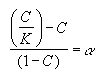# Use Empirical Alpha-Factors

#### Use Empirical Alpha-Factors

To utilize empirically determined Alpha-Factor terms, simply edit the EMPFAC.DAT file in the Probe for EPMA program data directory (usually C: \ProgramData\Probe Software\Probe for EPMA). If an Alpha-factor entry in the file matches the run conditions of the current run, it can be used to overwrite the previously internally calculated alpha-factor terms. The format of the EMPFAC.DAT file is described below :

52.5  20.0  1  "au"  "la"  "ag"  1.142   0.000   0.000 "NBS Spec. Pub. 260-28"

52.5  20.0  1  "ag"  "la"  "au"  1.483   0.000   0.000 "NBS Spec. Pub. 260-28"

52.5  20.0  1  "au"  "la"  "cu"  1.347   0.000   0.000 "NBS Spec. Pub. 260-28"

52.5  20.0  1  "cu"  "ka"  "au"  0.758   0.000   0.000 "NBS Spec. Pub. 260-28"

52.5  20.0  2  "au"  "la"  "ag"  1.159  -0.056   0.000 "NBS Spec. Pub. 260-28"

52.5  20.0  2  "ag"  "la"  "au"  1.505  -0.071   0.000 "NBS Spec. Pub. 260-28"

52.5  20.0  2  "au"  "la"  "cu"  1.347  -0.014   0.000 "NBS Spec. Pub. 260-28"

52.5  20.0  2  "cu"  "ka"  "au"  0.759  -0.007   0.000 "NBS Spec. Pub. 260-28"

52.5  20.0  3  "au"  "la"  "ag"  1.121   0.127  -0.179 "NBS Spec. Pub. 260-28"

52.5  20.0  3  "ag"  "la"  "au"  1.458   0.170  -0.247 "NBS Spec. Pub. 260-28"

52.5  20.0  3  "au"  "la"  "cu"  1.332   0.061  -0.075 "NBS Spec. Pub. 260-28"

52.5  20.0  3  "cu"  "ka"  "au"  0.740   0.089  -0.096 "NBS Spec. Pub. 260-28"

Each line contains a single empirical alpha-factors fit for half of a binary pair (one emitter-absorber system per line) for various take-off angles, operating voltage, elements, x-ray lines, etc.

Columns 1 and 2 contain the takeoff angle (degrees) and operating voltage (keV) that the alpha-factor was measured at. Column 3 is the fit flag (1 = constant expression, 2 = linear fit and 3 = polynomial fit). Columns 4 and 5 are the emitter element symbols and x-ray lines. Column 6 is the absorber element symbols. Note that Probe for EPMA only supports the use of elemental alpha-factors, i.e., referenced to elemental end-members as opposed to oxide end-members.

The three remaining columns are the alpha-factor terms. Note that constant alpha-factors have only one term, linear alpha-factors have two terms and non-linear or polynomial fit alpha-factors have three terms. For the non-linear alpha-factors, the first term is the intercept, the second term is the slope and the third term is the curvature term.

Empirical alpha-factors are calculated from experimentally measured k-ratios using a set of known binary (two element) standards covering a range of composition, by fitting the concentrations and intensity data to a plot of α vs. C, where C represents the standard concentrations and α is defined by the following expression :Each binary data set can be fit to any or all of the alpha-factor fits, that is, constant (single term at 50/50 composition), linear (two terms) or polynomial (three terms).